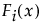Tip: Select the Comparison Criterion option to change the criterion for finding the best distribution.
 ‒ The option does not fit DS or TH distributions.
 ‒ If the data have negative values, then the option produces no results.
 ‒
whereis one of the supported distributions, k is the number of components in the mixture, and the wi are positive weights that sum to 1. The Fit Mixture option attempts to identify clusters of observations that are drawn from each of the component distributions, Fi(x). It estimates the parameters of the mixture and the probability that an observation is drawn from any given component.
 • Single Cluster assumes that all observations are affected by all of the ingredient distributions to some extent. None of the densities stand out as affecting only a portion of the observations.
 • Separable Clusters assumes that the ingredient distributions affect some observations more profoundly than others. For separable clusters, each of the k densities has an identifiable mode and defines a cluster.
 • Overlapping Clusters assumes a situation that is intermediate between Single Cluster and Separable Clusters. Some densities stand out, but others jointly affect a portion of the observations. In this case, there are m clusters in the data, where m is less than k, the total number of densities.
 1 Clusters of observations are defined.
 2 Assignment of clusters to densities is based on the Starting Value Method:
 ‒ For Separable Clusters, the highest likelihood assignment of clusters to the specified ingredient densities is determined by examining the possible permutations.
 ‒ For Overlapping Clusters, the highest likelihood assignment of clusters to the specified ingredient densities is determined by examining the possible permutations of clusters and combinations of observations.
Click Go to fit the desired mixture. The Model List is updated with the model that you fit, and a report with the name of the mixture model is added.
 • Smaller values of each of these statistics indicate a better fit.
 • The rows are sorted by AICc.
 • The Comparison Criterion red triangle option does not affect the order of models in the Model List.
 •
Parameter estimates are given for each distribution in the mixture. The Parameter column also includes parameters called Portion <i>, where i = 1, 2, .., k-1. These are estimates of the weights wi for the mixture. Since the weights sum to 1, the kth weight can be computed from the first k - 1 weights.
 ‒ The Distribution Profiler shows cumulative failure probability as a function of time.
 ‒ The Quantile Profiler shows failure time as a function of cumulative probability.
 ‒ The Hazard Profiler shows the hazard rate as a function of time.
 ‒ The Density Profiler shows the density function for the distribution.
 1 Select Help > Sample Data Library and open Reliability/Mixture Demo.jmp.
 2 Select Analyze > Reliability and Survival > Life Distribution.
 3 Select Y1 and click Y, Time to Event.
 4 Click OK.
 5 Select Fit Mixture from the red triangle menu next to Life Distribution.
 6 Type 2 in the Quantity box next to Weibull.
 7 Select Separable Clusters in the Starting Value Methods panel.
 8 Click Go.
Fit Mixture for Weibull (2)
 9 Type 1 next to Lognormal and 1 next to Weibull.
 10 Click Go.
Fit Mixture for Lognormal(1), Weibull(1)
 1 From the Lognormal(1), Weibull(1) red triangle menu, select Save Predictions.
 ‒ Lognormal(1), Weibull(1) - Predicted Probability from Lognormal
 ‒ Lognormal(1), Weibull(1) - Predicted Probability from Weibull
 2 Select Analyze > Distribution.
 3
 4 Check Histograms Only.
 5 Click OK.
 6 In the histogram for Lognormal(1), Weibull(1) - Predicted Probability from Weibull, click in the bar corresponding to the value near 1.
Histograms for Mixture Probabilities
whererepresents the cumulative failure distributions for the ith risk and k is the number of components (or risks) in the mixture. The Fit Competing Risk Mixture option attempts to identify clusters of observations that are drawn from each of the component distributions, Fi(x). It estimates the parameters of the mixture and the probability that an observation is drawn from any given component.
The Competing Risk Mixture report is structured in a fashion that mirrors the Mixture report. See Mixture. However, the Fit Competing Risk Mixture reports do not show a Density Overlay plot. They show a Distribution Overlay plot instead.